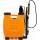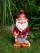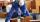# Strawberries

Father collects strawberries himself in 4 hours, son in 14 hours. How long will it take them along when dad comes to help son collect strawberries after 3 hours?

Result

x =  2.444 h

#### Solution:

x/4+(x+3)/14=1

9x = 22

x = 229 ≈ 2.444444

Calculated by our simple equation calculator.

Leave us a comment of example and its solution (i.e. if it is still somewhat unclear...):Be the first to comment!#### To solve this example are needed these knowledge from mathematics:

Need help calculate sum, simplify or multiply fractions? Try our fraction calculator. Do you have a linear equation or system of equations and looking for its solution? Or do you have quadratic equation?

## Next similar examples:

1. NovakNovak needed to dig up three of the same pit in the garden. The first pit dug father alone for 15 hours. His second dig son helped him and it did that in six hours. The third pit dug son himself. How long it took him?
2. TogetherGrandfather, father, and son are carpenters. They decided to make a wardrobe. Father produces it for 12 hours, his son for 15 hours, and grandfather for 10 hours. How long will they make it together?
3. SprayersThe vineyard can be sprayed first sprayer in 5 hours and with the second in 8 hours. How long would it take if we use sprayers both at once?
4. Pumps A and BPump A fill the tank for 12 minutes, pump B for 24 minutes. How long will take to fill the tank if he is only three minutes works A and then both pumps A and B?For the construction of the road dam, it is supposed to transport six trucks a total of 18 days. After three days, two more cars were sent to the construction site. How long will it take to carry the material?
6. PlayingHow long have we trained on the pitch when we know that the warm-up took 10 minutes, we trained passes for one-third of the time and we played football half the time?
7. Six yearsIn six years Jan will be twice as old as he was six years ago. How old is he?
8. UN 1If we add to an unknown number his quarter, we get 210. Identify unknown number.
9. Unknown numberI think the number - its sixth is 3 smaller than its third.
10. Cleaning windowsCleaning company has to wash all the windows of the school. The first day washes one-sixth of the windows of the school, the next day three more windows than the first day and the remaining 18 windows washes on the third day. Calculate how many windows ha
11. FractionsThree-quarters of an unknown number are 4/5. What is 5/6 of this unknown number?
12. Simple equationSolve for x: 3(x + 2) = x - 18
13. EqnSolve equation with fractions: 2x/3-50=40+x/4
14. Simple equation 6Solve equation with one variable: X/2+X/3+X/4=X+4
15. DwarfsFour dwarfs would prepare firewood for Snow White in580 minutes. After an hour and a half they recruit friends so that finished preparing the wood for 280 minutes. How many dwarfs they recruited?
16. Unknown numberIdentify unknown number which 1/5 is 40 greater than one tenth of that number.
17. Bricklayers8 bricklayers build a house for 630 days. How many bricklayers have to add after 150 days, so then the whole building completed in (next) 320 days?